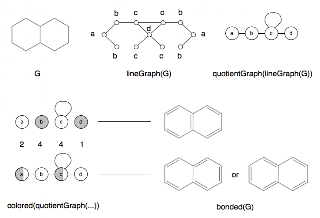Skip to main content

### Line Graphs and Double Bonding Systems

After looking at a CDK tool for fixing bond orders for aromatic systems (DeduceBondSystemTool in the smiles package) I wondered if there was a more general approach. That is, the problem is to take a molecular graph with no double bonds and generate all possible double bonded systems.

One possibility might be to first convert the graph (G) into a form known as a line graph (lg(G)) where every vertex in lg(G) is an edge in G. If these vertices are labelled to represent the bond order, then an aromatic system has a particular line graph. For example, here is benzene:
The dashed lines show the construction of the line graph, and the labels '-' and '=' mean single and double. Now obviously, the two resulting graphs are essentially the same, so it would be nice to remove this redundancy. An example of two different bonding systems comes from phenanthrene:Which is great, but how to generate all non-redundant colorings of the line graphs? Since a line graph is just a graph, it can have a signature, and a signature quotient graph. This can then be colored:However, in this example it is necessary to 'half-color' some of the vertices of the quotient graph ... which doesn't quite seem to work. The numbers in between the colored quotient graphs show how many line graph vertices are in each of the symmetry classes.

In any case, this is a bit of a toy problem, with only a partial solution, but here is a code repository for a sketch of the code. Note that the algorithm is missing!

### How many isomers of C4H11N are there?

One of the most popular queries that lands people at this blog is about the isomers of C4H11N - which I suspect may be some kind of organic chemistry question on student homework. In any case, this post will describe how to find all members of a small space like this by hand rather than using software.

Firstly, lets connect all the hydrogens to the heavy atoms (C and N, in this case). For example:

Now eleven hydrogens can be distributed among these five heavy atoms in various ways. In fact this is the problem of partitioning a number into a list of other numbers which I've talked about before. These partitions and (possible) fragment lists are shown here:

One thing to notice is that all partitions have to have 5 parts - even if one of those parts is 0. That's not strictly a partition anymore, but never mind. The other important point is that some of the partitions lead to multiple fragment lists - [3, 3, 2, 2, 1] could have a CH+NH2 or an NH+CH2.

The final step is to connect u…

### The Gale-Ryser Theorem

This is a small aside. While reading a paper by Grüner, Laue, and Meringer on generation by homomorphism they mentioned the Gale-Ryser (GR) theorem. As it turns out, this is a nice small theorem closely related to the better known Erdős-Gallai (EG).

So, GR says that given two partitions of an integer (p and q) there exists a (0, 1) matrixA iff p*dominatesq such that the row sum vector r(A) = p and the column sum vector c(A) = q.

As with most mathematics, that's quite terse and full of terminology like 'dominates' : but it's relatively simple. Here is an example:

The partitions p and q are at the top left, they both sum to 10. Next, p is transposed to get p* = [5, 4, 1] and this is compared to q at the bottom left. Since the sum at each point in the sequence is greater (or equal) for p* than q, the former dominates. One possible matrix is at the top left with the row sum vector to the right, and the column sum vector below.

Finally, the matrix can be interpreted as a bi…

### Havel-Hakimi Algorithm for Generating Graphs from Degree Sequences

A degree sequence is an ordered list of degrees for the vertices of a graph. For example, here are some graphs and their degree sequences:

Clearly, each graph has only one degree sequence, but the reverse is not true - one degree sequence can correspond to many graphs. Finally, an ordered sequence of numbers (d1 >= d2 >= ... >= dn > 0) may not be the degree sequence of a graph - in other words, it is not graphical.

The Havel-Hakimi (HH) theorem gives us a way to test a degree sequence to see if it is graphical or not. As a side-effect, a graph is produced that realises the sequence. Note that it only produces one graph, not all of them. It proceeds by attaching the first vertex of highest degree to the next set of high-degree vertices. If there are none left to attach to, it has either used up all the sequence to produce a graph, or the sequence was not graphical.

The image above shows the HH algorithm at work on the sequence [3, 3, 2, 2, 1, 1]. Unfortunately, this produce…